# A parallel-plate air-filled capacitor having area 31 cm2 and plate spacing 3.0 mm is charged to...

A parallel-plate air-filled capacitor having area 31 cm2 and plate spacing 3.0 mm is charged to a potential difference of 400 V. Find the following values.

(a) the capacitance

pF

(b) the magnitude of the charge on each plate

nC

(c) the stored energy

μJ

(d) the electric field between the plates

V/m

(e) the energy density between the plates

J/m3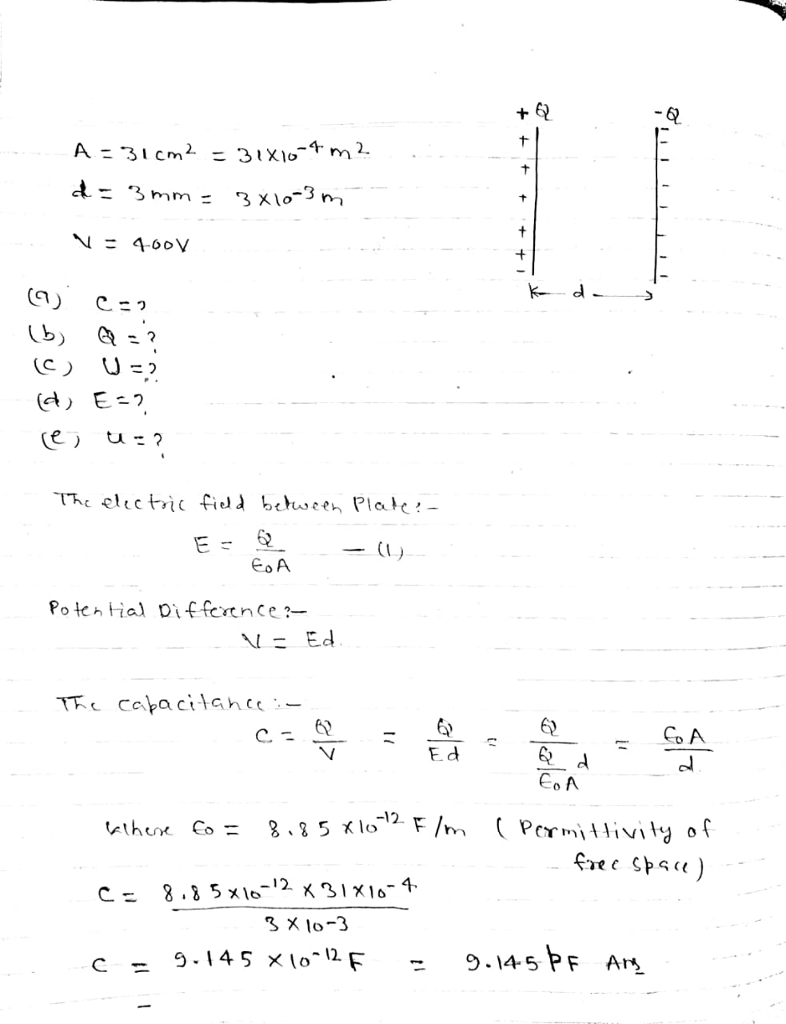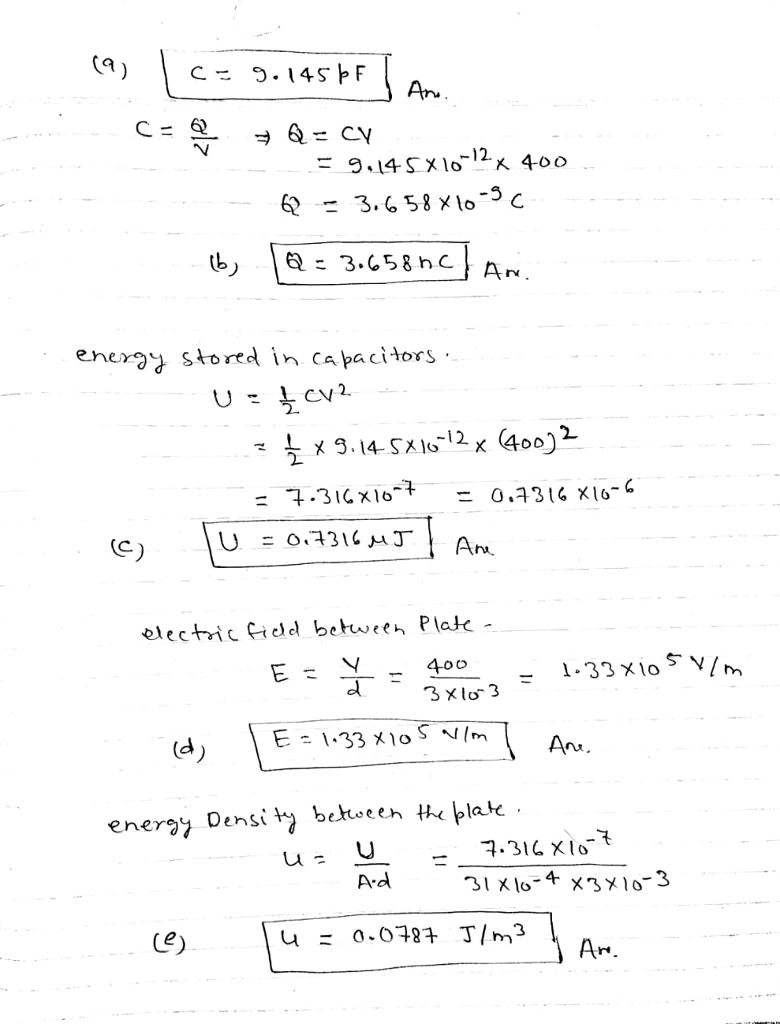#### Earn Coin

Coins can be redeemed for fabulous gifts.

Similar Homework Help Questions
• ### A parallel-plate air-filled capacitor having area 44 cm2 and plate spacing 5.0 mm is charged to...

A parallel-plate air-filled capacitor having area 44 cm2 and plate spacing 5.0 mm is charged to a potential difference of 850 V. Find the following values. (a) the capacitance (pF) (b) the magnitude of the charge on each plate (nC) (c) the stored energy (μJ) (d) the electric field between the plates (V/m) (e) the energy density between the plates (J/m3)

• ### A parallel-plate air-filled capacitor having area 40 cm2 and plate spacing 1.5 mm is charged to...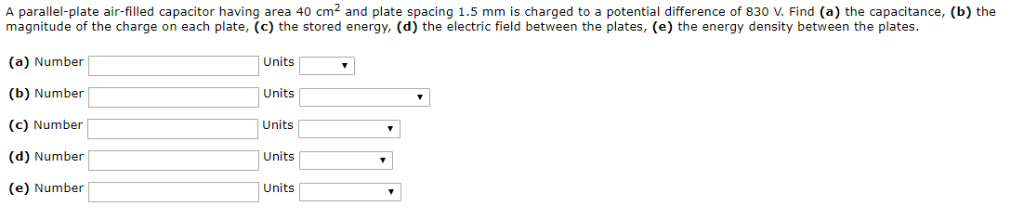A parallel-plate air-filled capacitor having area 40 cm2 and plate spacing 1.5 mm is charged to a potential difference of 830 V. Find (a) the capacitance, (b) the 2 9 5 8 0 magnitude of the charge on each plate, (c) the stored energy, (d) the electric field between the plates, (e) the energy density between the plates (a) Number (b) Number (c) Number (d) Number (e) Number Units Units Units Units Units

• ### Chapter 25, Problem 032 A parallel-plate air-filled capacitor having area 48 cm2 and plate spacing 1.2...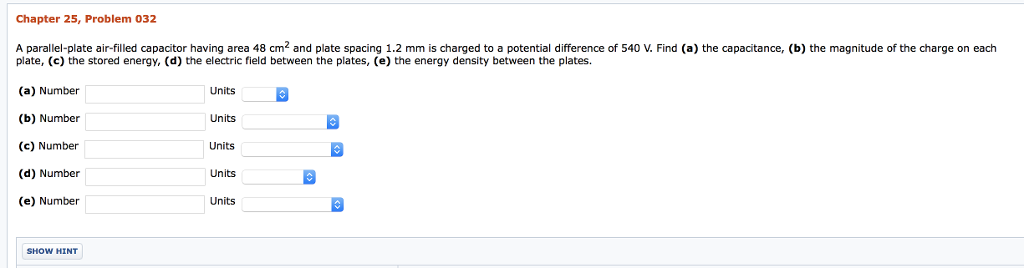Chapter 25, Problem 032 A parallel-plate air-filled capacitor having area 48 cm2 and plate spacing 1.2 mm is charged to a potential difference of 540 V. Find (a) the capacitance, (b) the magnitude of the charge on each plate, (c) the stored energy, (d) the electric field between the plates, (e) the energy density between the plates. (a) Number (b) Number () Number (d) Number (e) Number Units Units Units Units Units SHOW HINT

• ### Chapter 25, Problem 032 A parallel-plate air-filled capacitor having area 46 cm2 and plate spacing 1.2...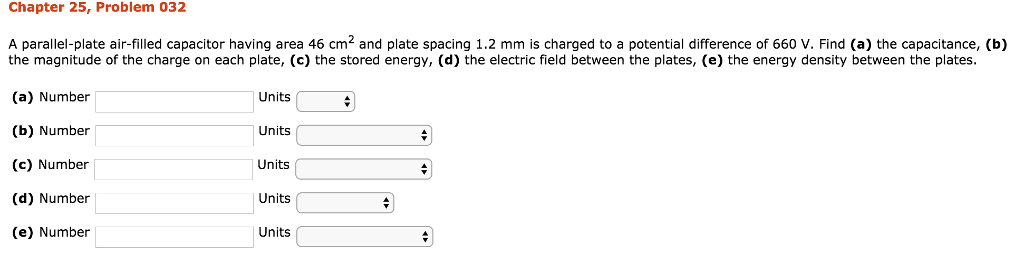Chapter 25, Problem 032 A parallel-plate air-filled capacitor having area 46 cm2 and plate spacing 1.2 mm is charged to a potential difference of 660 V. Find (a) the capacitance, (b) 9 the magnitude of the charge on each plate, (c) the stored energy, (d) the electric field between the plates, (e) the energy density between the plates. (a) Number (b) Number (c) Number (d) Number (e) Number Units Units Units Units Units

• ### Chapter 25, Problem 032 A parallel-plate air-filled capacitor having area 50 cm2 and plate spacing 0.82...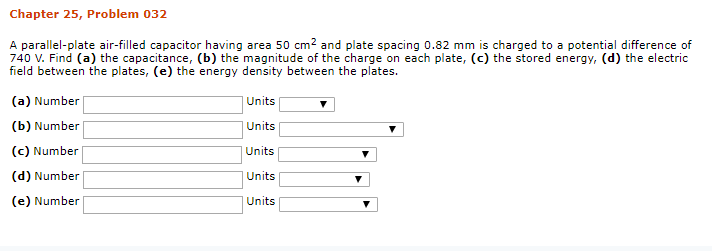Chapter 25, Problem 032 A parallel-plate air-filled capacitor having area 50 cm2 and plate spacing 0.82 mm is charged to a potential difference of 740 V. Find (a) the capacitance, (b) the magnitude of the charge on each plate, (c) the stored energy, (d) the electric field between the plates, (e) the energy density between the plates. (a) Number (b) Number (c) Number (d) Number (e) Number Units Units Units Units Uni

• ### An air-filled capacitor consists of two parallel plates, each with an area of 7.60 cm2, separated...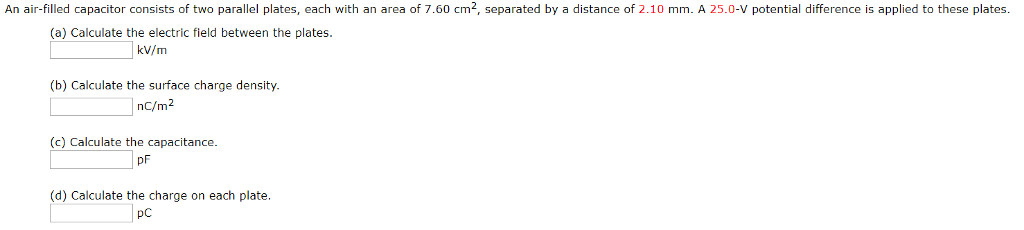An air-filled capacitor consists of two parallel plates, each with an area of 7.60 cm2, separated by a distance of 2.10 mm. A 25.0-V potential difference is applied to these plates (a) Calculate the electric field between the plates kV/m (b) Calculate the surface charge density. nc/m2 (c) Calculate the capacitance. pF (d) Calculate the charge on each plate pc

• ### An air-filled capacitor consists of two parallel plates, each with an area of 7.60 cm2, separated...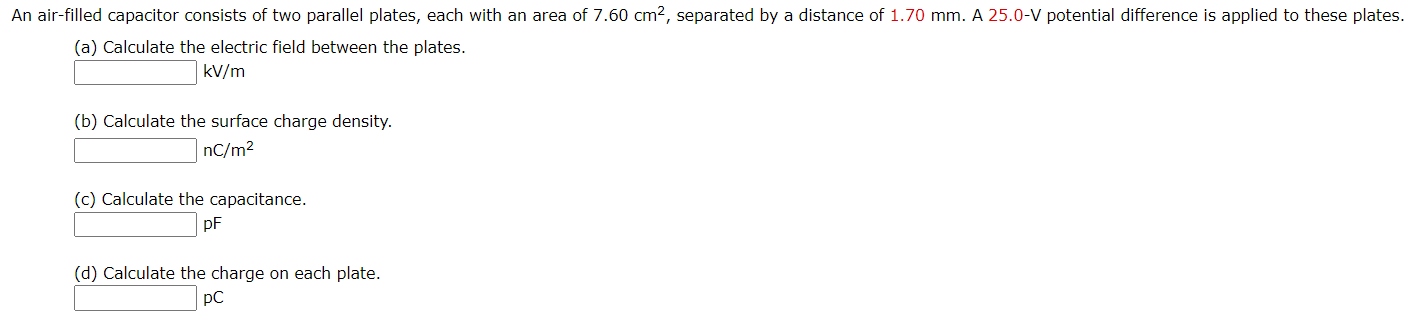An air-filled capacitor consists of two parallel plates, each with an area of 7.60 cm2, separated by a distance of 1.70 mm. A 25.0-V potential difference is applied to these plates. (a) Calculate the electric field between the plates. kV/m (b) Calculate the surface charge density. nC/m2 (c) Calculate the capacitance. pF (d) Calculate the charge on each plate. pC

• ### The parallel plates in a capacitor, with a plate area of 9.00 cm2 and an air-filled...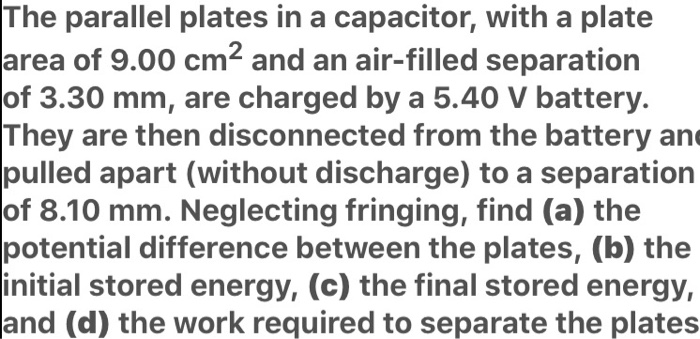The parallel plates in a capacitor, with a plate area of 9.00 cm2 and an air-filled separation of 3.30 mm, are charged by a 5.40 V battery. They are then disconnected from the battery and pulled apart (without discharge) to a separation of 8.10 mm. Neglecting fringing, find (a) the potential difference between the plates, (b) the initial stored energy, (c) the final stored energy, and (d) the work required to separate the plates

• ### The parallel plates in a capacitor, with a plate area of 9.90 cm2 and an air-filled...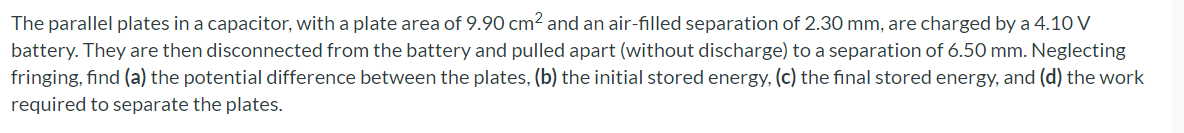The parallel plates in a capacitor, with a plate area of 9.90 cm2 and an air-filled separation of 2.30 mm, are charged by a 4.10 V battery. They are then disconnected from the battery and pulled apart (without discharge) to a separation of 6.50 mm. Neglecting fringing, find (a) the potential difference between the plates, (b) the initial stored energy, (c) the final stored energy, and (d) the work required to separate the plates.

• ### The parallel plates in a capacitor, with a plate area of 9.00 cm2 and an air-filled...

The parallel plates in a capacitor, with a plate area of 9.00 cm2 and an air-filled separation of 3.30 mm, are charged by a 5.40 V battery. They are then disconnected from the battery and pulled apart (without discharge) to a separation of 8.10 mm. Neglecting fringing, find (a) the potential difference between the plates, (b) the initial stored energy, (c) the final stored energy, and (d) the work required to separate the plates.

Free Homework App